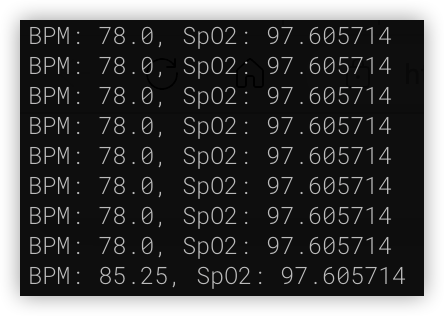# Demo 7: The Use of Heart Rate Sensors on VisionFive 2

According to StarFive, developers have successfully used heart rate sensor (MAX30102) on VisionFive/VisionFive 2. The specific instructions are as follows:

1. Preparation

2. The principle of heart rate sensor
The specific heart rate sensor modules used in this demo are as follows:

MAX30102 is a sensor module integrated with pulse oximeter and heart rate monitoring. The module integrates red light/infrared LED light source, which converts the light transmission rate change signal during blood vessel pulsation into electrical signal acquisition. This module uses 5V/3.3V power supply, using I2C interface for communication.

4. The use of heart rate sensor

Firstly, refer to the following figure and connect the heart rate sensor module to VisionFive:

connection

Note:
The power supply voltage should be determined based on the actual sensor used. The sensor module used in this demo uses a 5V power supply voltage.

After reading RAW data from MAX30102, conversion is required to express the actual measured pulse blood oxygen content and heart rate. We can download third-party libraries to complete these tasks.

``````git clone git@github.com:doug-burrell/max30102.git

pip install numpy
pip install smbus
``````

Then write the following program:

``````# -*- coding: utf-8 -*-

from max30102 import MAX30102
import numpy as np
import hrcalc
import time

# Initialize MAX30102 sensor
sensor = MAX30102()

# Cache
ir_data = []
red_data = []
bpms = []

# Output result setting
print_raw = False
print_result = True

while True:
num_bytes = sensor.get_data_present()
if num_bytes > 0:
# Parsing data
while num_bytes > 0:
num_bytes -= 1
ir_data.append(ir)
red_data.append(red)
if print_raw:
print("{0}, {1}".format(ir, red))

while len(ir_data) > 100:
ir_data.pop(0)
red_data.pop(0)

if len(ir_data) == 100:
# Calculate data
bpm, valid_bpm, spo2, valid_spo2 = hrcalc.calc_hr_and_spo2(ir_data, red_data)
if valid_bpm:
bpms.append(bpm)
while len(bpms) > 4:
bpms.pop(0)
bpm = np.mean(bpms)
if (np.mean(ir_data) < 50000 and np.mean(red_data) < 50000):
bpm = 0
if print_result:
print("No fingers detected")
if print_result and bpm>0 and spo2>0:
print("BPM: {0}, SpO2: {1}".format(bpm, spo2))

time.sleep(0.01)

# Turn off detection will turn off the LED and reduce power consumption
sensor.shutdown()
``````

The logic of the above program is to first read a certain amount of data, then decode the data, and finally calculate the actual measurement results and output.
After writing, run read_max30102.py. After the measured LED lights up, place your finger above the LED and observe the output result.

``````pyhon3 read_max30102.py
``````

The actual operation results are as follows:
When the measurement is activated, the LED light on the MAX30102 sensor will light up:

Place your finger on the LED and after a period of time, the detection result will be displayed:In the above output record, BPM represents heart rate data and SpO2 represents the percentage of blood oxygen concentration.
In actual measurements, the resulting values may drift to a certain extent.
When used in real situations, it is often necessary to continuously read data for a period of time, and then use certain algorithms to obtain the final reasonable test results.

4. Summary
In this demo, we learned the basic use of heart rate sensors.
Heart rate sensor is widely used in health detection situations, and most mainstream smart bracelets and watches currently use it for health detection.

Author: @HonestQiao

2 Likes